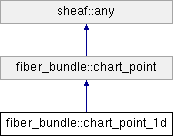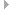SheafSystem  0.0.0.0
fiber_bundle::chart_point_1d Class Reference

A point in a 1D chart space. More...

`#include <chart_point_1d.h>`

Inheritance diagram for fiber_bundle::chart_point_1d:## CHART_POINT_1D FACET

coord_type _local_coords 
The local coordinates of this point. More...

chart_point_1d ()
Default constructor. More...

chart_point_1d (const chart_point_1d &xother)
Copy constructor. More...

virtual ~chart_point_1d ()
Destructor. More...

chart_point_1d (pod_index_type xchart_id, coord_type xu)
Creates an instance with chart_id() == xchart_id, u() == xu. More...

chart_point_1d (const scoped_index &xchart_id, coord_type xu)
Creates an instance with chart_id() == xchart.hub_pod(), u() == xu. More...

chart_point_1d (pod_index_type xchart_id, const coord_type *xlocal_coords, size_type xlocal_coords_ub)
Creates an instance with chart_id() == xchart_id and local_coord(i) == xlocal_coords[i]. More...

chart_point_1d (const scoped_index &xchart_id, const coord_type *xlocal_coords, size_type xlocal_coords_ub)
Creates an instance with chart_id() == xchart_id.hub_pod() and local_coord(i) == xlocal_coords[i]. More...

virtual int db () const
The dimension of this chart. More...

virtual coord_type local_coord (int xi) const
The xi-th local coordinate of this point. More...

virtual void put_local_coord (int xi, coord_type xvalue)
Sets the xi-th local coordinate of this point to xvalue. More...

coord_typelocal_coords ()
The array of local coordinates. More...

const coord_typelocal_coords () const
The array of local coordinates, const version. More...

coord_typeu ()
The 0-th (and only) local coordinate of this; mutable version. More...

const coord_typeu () const
The 0-th (and only) local coordinate of this; const version. More...

void put (pod_index_type xchart_id, coord_type xu)
Sets chart_id() == xchart_id, u() == xu. More...

void put (const scoped_index &xchart_id, coord_type xu)
Sets chart_id() == xchart_id.hub_pod(), u() == xu. More...

## ANY FACET

virtual chart_point_1dclone () const
Virtual constructor, makes a new instance of the same type as this. More...

virtual bool invariant () const
Class invariant. More...

virtual bool is_ancestor_of (const any *other) const
Conformance test; true if other conforms to this. More...

chart_point_1doperator= (const chart_point &xother)
Assignment operator. More...

chart_point_1doperator= (const chart_point_1d &xother)
Assignment operator. More...Public Types inherited from fiber_bundle::chart_point
typedef chart_point_coord_type coord_type
The type of local coordinate; the scalar type for the local coordinate vector space. More...Public Member Functions inherited from fiber_bundle::chart_point
virtual ~chart_point ()
Destructor. More...

pod_index_type chart_id () const
The id of the chart this point is in. More...

void put_chart_id (pod_index_type xchart)
Sets chart_id() to xchart_id. More...

void put_chart_id (const scoped_index &xchart)
Sets chart_id() to xchart_id.hub_pod(). More...

virtual void put_local_coord (int xi, coord_type xvalue)=0
Sets the xi-th local coordinate of this point to xvalue. More...

void put_local_coords (const coord_type *xvalues, size_type xvalues_ub)
Sets the local coordinates to the values in xvalues. More...

void put_local_coords_zero ()
Sets the local coordinates to the zero vector. More...

bool is_valid () const
True if this ia a valid point in a chart. More...

void invalidate ()
Makes this invalid. More...

chart_pointoperator= (const chart_point &xother)
Assignment operator. More...

bool operator== (const chart_point &xother) const
Equality operator. More...

void to_stream (std::ostream &xos=std::cout) const
Write instance information to an ostream (default = stdout). More...

std::string to_string () const
Get instance information as a string. More...Public Member Functions inherited from sheaf::any
bool is_same_type (const any *other) const
True if other is the same type as this. More...

virtual ~any ()
Destructor. More...

bool invariant_check () const
True if invariant checking is enabled. More...

void enable_invariant_check () const
Enable invariant checking. More...

void disable_invariant_check () const
Disable invariant check. Intended for preventing recursive calls to invariant and for suppressing invariant checking during multi-phase initialization. More...

int disable_invariance_check_request_depth () const
Number of times disable_invariant_check has been called without matching call to enable_invariant_check. More...Static Public Member Functions inherited from fiber_bundle::chart_point
static chart_pointnew_chart_point (int xdb)
Factory method; creates a chart point with db() == xdb. More...Protected Member Functions inherited from fiber_bundle::chart_point
chart_point ()
Default constructor. More...Protected Member Functions inherited from sheaf::any
any ()
default constructor More...Protected Attributes inherited from fiber_bundle::chart_point
pod_index_type _chart_id
The id of the chart this point is in. More...

## Detailed Description

A point in a 1D chart space.

Definition at line 40 of file chart_point_1d.h.

## ◆ chart_point_1d() [1/6]

 fiber_bundle::chart_point_1d::chart_point_1d ( )

Default constructor.

Postcondition
• !is_valid()

Definition at line 33 of file chart_point_1d.cc.

References sheaf::invalid_pod_index(), and sheaf::is_valid().

Referenced by ~chart_point_1d().

## ◆ chart_point_1d() [2/6]

 fiber_bundle::chart_point_1d::chart_point_1d ( const chart_point_1d & xother )

Copy constructor.

Postcondition
• *this == xother

Definition at line 52 of file chart_point_1d.cc.

References ~chart_point_1d().

## ◆ ~chart_point_1d()

 fiber_bundle::chart_point_1d::~chart_point_1d ( )
virtual

Destructor.

Definition at line 71 of file chart_point_1d.cc.

Referenced by chart_point_1d().

## ◆ chart_point_1d() [3/6]

 fiber_bundle::chart_point_1d::chart_point_1d ( pod_index_type xchart_id, coord_type xu )

Creates an instance with chart_id() == xchart_id, u() == xu.

## ◆ chart_point_1d() [4/6]

 fiber_bundle::chart_point_1d::chart_point_1d ( const scoped_index & xchart_id, coord_type xu )

Creates an instance with chart_id() == xchart.hub_pod(), u() == xu.

## ◆ chart_point_1d() [5/6]

 fiber_bundle::chart_point_1d::chart_point_1d ( pod_index_type xchart_id, const coord_type * xlocal_coords, size_type xlocal_coords_ub )

Creates an instance with chart_id() == xchart_id and local_coord(i) == xlocal_coords[i].

## ◆ chart_point_1d() [6/6]

 fiber_bundle::chart_point_1d::chart_point_1d ( const scoped_index & xchart_id, const coord_type * xlocal_coords, size_type xlocal_coords_ub )

Creates an instance with chart_id() == xchart_id.hub_pod() and local_coord(i) == xlocal_coords[i].

## ◆ clone()

 fiber_bundle::chart_point_1d * fiber_bundle::chart_point_1d::clone ( ) const
virtual

Virtual constructor, makes a new instance of the same type as this.

Postcondition
• result->is_same_type(this)

Implements fiber_bundle::chart_point.

Definition at line 405 of file chart_point_1d.cc.

References invariant(), and sheaf::any::is_same_type().

Referenced by u().

## ◆ db()

 int fiber_bundle::chart_point_1d::db ( ) const
virtual

The dimension of this chart.

Postcondition
• result == 1

Implements fiber_bundle::chart_point.

Definition at line 182 of file chart_point_1d.cc.

References local_coord().

Referenced by ~chart_point_1d().

## ◆ invariant()

 bool fiber_bundle::chart_point_1d::invariant ( ) const
virtual

Class invariant.

Invariant

Reimplemented from fiber_bundle::chart_point.

Definition at line 426 of file chart_point_1d.cc.

References fiber_bundle::chart_point::invariant(), and is_ancestor_of().

Referenced by clone().

## ◆ is_ancestor_of()

 bool fiber_bundle::chart_point_1d::is_ancestor_of ( const any * other ) const
virtual

Conformance test; true if other conforms to this.

Precondition
• other != 0

Reimplemented from fiber_bundle::chart_point.

Definition at line 457 of file chart_point_1d.cc.

References operator=().

Referenced by invariant().

## ◆ local_coord()

 fiber_bundle::chart_point_1d::coord_type fiber_bundle::chart_point_1d::local_coord ( int xi ) const
virtual

The xi-th local coordinate of this point.

Precondition
• (0 <= xi) && (xi < db())
Todo:
uncomment when invariant changed to invariant() const; ensure(invariant());

Implements fiber_bundle::chart_point.

Definition at line 204 of file chart_point_1d.cc.

References put_local_coord().

Referenced by db().

## ◆ local_coords() [1/2]

 fiber_bundle::chart_point_1d::coord_type * fiber_bundle::chart_point_1d::local_coords ( )
virtual

The array of local coordinates.

Postcondition
• result != 0

Implements fiber_bundle::chart_point.

Definition at line 252 of file chart_point_1d.cc.

Referenced by put_local_coord().

## ◆ local_coords() [2/2]

 const fiber_bundle::chart_point_1d::coord_type * fiber_bundle::chart_point_1d::local_coords ( ) const
virtual

The array of local coordinates, const version.

Postcondition
• result != 0

Implements fiber_bundle::chart_point.

Definition at line 274 of file chart_point_1d.cc.

References u().

## ◆ operator=() [1/2]

 chart_point_1d& fiber_bundle::chart_point_1d::operator= ( const chart_point & xother )
inline

Assignment operator.

Definition at line 187 of file chart_point_1d.h.

References fiber_bundle::chart_point::operator=().

Referenced by is_ancestor_of().

## ◆ operator=() [2/2]

 fiber_bundle::chart_point_1d & fiber_bundle::chart_point_1d::operator= ( const chart_point_1d & xother )

Assignment operator.

Postcondition
• -unexecutable( *this == xother )

Definition at line 478 of file chart_point_1d.cc.

References fiber_bundle::chart_point::_chart_id, and _local_coords.

## ◆ put() [1/2]

 void fiber_bundle::chart_point_1d::put ( pod_index_type xchart_id, coord_type xu )

Sets chart_id() == xchart_id, u() == xu.

Referenced by u().

## ◆ put() [2/2]

 void fiber_bundle::chart_point_1d::put ( const scoped_index & xchart_id, coord_type xu )

Sets chart_id() == xchart_id.hub_pod(), u() == xu.

## ◆ put_local_coord()

 void fiber_bundle::chart_point_1d::put_local_coord ( int xi, coord_type xvalue )
virtual

Sets the xi-th local coordinate of this point to xvalue.

Precondition
• (0 <= xi) && (xi < db())
Todo:
uncomment when invariant changed to invariant() const; ensure(invariant());
Postcondition
• isunordered_or_equals(local_coord(xi), xvalue)

Definition at line 228 of file chart_point_1d.cc.

References sheaf::isunordered_or_equals(), and local_coords().

Referenced by local_coord().

## ◆ u() [1/2]

 fiber_bundle::chart_point_1d::coord_type & fiber_bundle::chart_point_1d::u ( )

The 0-th (and only) local coordinate of this; mutable version.

Todo:
uncomment when invariant changed to invariant() const; ensure(invariant());
Postcondition
• isunordered_or_equals(result, local_coord(0))

Definition at line 318 of file chart_point_1d.cc.

References clone(), sheaf::scoped_index::hub_pod(), sheaf::isunordered_or_equals(), and put().

Referenced by local_coords(), and u().

## ◆ u() [2/2]

 const fiber_bundle::chart_point_1d::coord_type & fiber_bundle::chart_point_1d::u ( ) const

The 0-th (and only) local coordinate of this; const version.

Todo:
uncomment when invariant changed to invariant() const; ensure(invariant());
Postcondition
• isunordered_or_equals(result, local_coord(0))

Definition at line 296 of file chart_point_1d.cc.

References sheaf::isunordered_or_equals(), and u().

## ◆ _local_coords

 coord_type fiber_bundle::chart_point_1d::_local_coords
protected

The local coordinates of this point.

Definition at line 141 of file chart_point_1d.h.

Referenced by operator=().

The documentation for this class was generated from the following files: